# Inference in Neural Networks using an Explainable Parameter Encoder Network

A Parameter Encoder Neural Network (PENN) is an explainable machine learning technique that solves two problems associated with traditional XAI algorithms:

1. It permits the calculation of local parameter distributions. Parameter distributions are often more interesting than feature contributions — particularly in economic and financial applications — since the parameters disentangle the effect from the observation (the contribution can roughly be defined as the demeaned product of effect and observation).
2. It solves a problem of biased contributions that is inherent to many traditional XAI algorithms. Particularly in the setting where neural networks are powerful — in interactive, dependent processes — traditional XAI can be biased, by attributing effect to each feature independently.

At the end of the tutorial, I will have estimated the following highly nonlinear parameter functions for a simulated regression with three variables: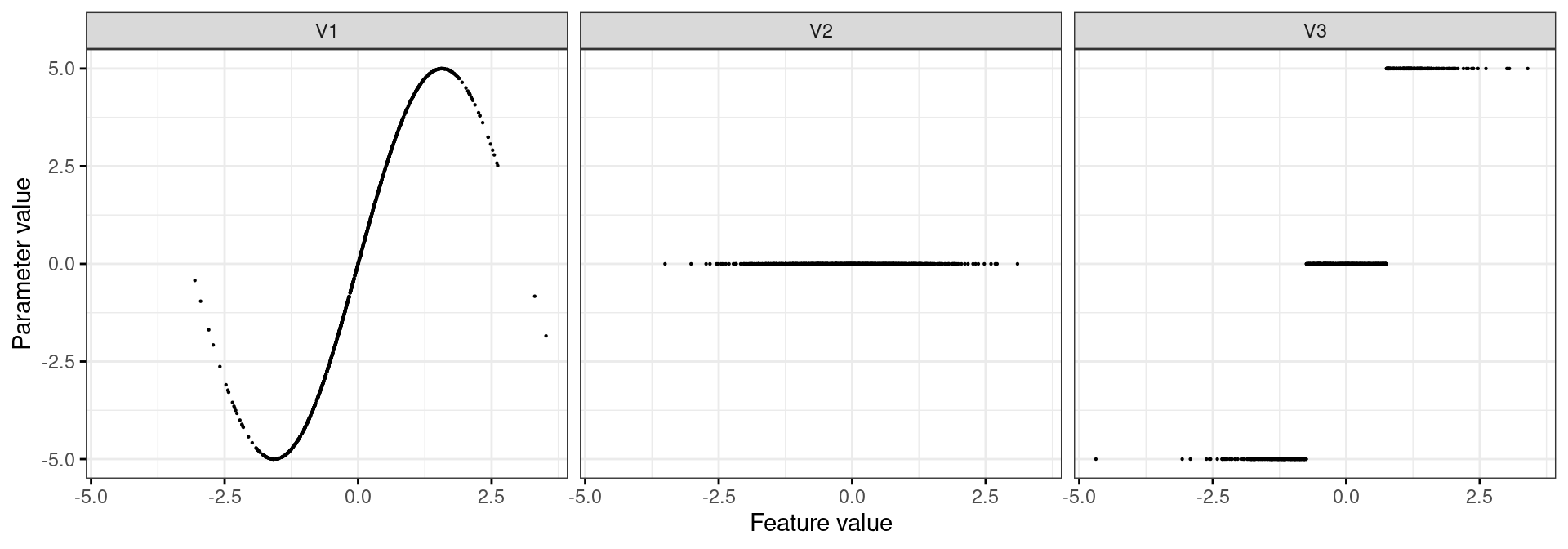A Github version of the code can be found here.

## A brief look at the maths

The PENN architecture consists of an inference network and a locally parameterized regression likelihood. The inference network is a generative neural network that uses input data to generate local parameter distributions for a locally-linear regression (or logistic regression). The local parameters are evaluated using the likelihood function and the network is trained using variational inference techniques that are well-known, for instance, from the field of variational autoencoders.

The problem of estimating local parameter distributions can be stated in the variational Bayesian setting as a minimization of the divergence (in this case the Kullback-Leibler Divergence) between and a true and an approximating parameter distribution:

$D_{KL}\left(q_{\boldsymbol{\theta}}(\boldsymbol{\beta}_i|\boldsymbol{x}_i) || p(\boldsymbol{\beta}_i|\boldsymbol{x}_i,y_i)\right) = -\int q_{\boldsymbol{\theta}}(\boldsymbol{\beta}_i|\boldsymbol{x}_i) \log \frac{p(\boldsymbol{\beta}_i|\boldsymbol{x}_i,y_i)}{q_{\boldsymbol{\theta}}(\boldsymbol{\beta}_i|\boldsymbol{x}_i)} d\boldsymbol{\beta}_i,$

where $$q_{\boldsymbol{\theta}}(\cdot)$$ is the approximating function, also called the inference network, and $$p(\cdot)$$ is the true parameter distribution.

Applying Bayes’ rule, summing over the observation dimension $$i$$, and moving terms around — the details of which can be found in … —, the above KL divergence yields an expectation lower bound (ELBO) that serves as the PENN loss function:

$\mathbb{E}_{\boldsymbol{\beta}\sim q_{\boldsymbol{\theta}}(\boldsymbol{\beta}|\boldsymbol{x})} \log p(y|\boldsymbol{\beta}, \boldsymbol{x}) - \sum_{i = 1}^N D_{KL}\left(q_{\boldsymbol{\theta}}(\boldsymbol{\beta}_i|\boldsymbol{x}_i) || p(\boldsymbol{\beta}_i|\boldsymbol{x}_i)\right).$

The ELBO contains the two elements discussed at the onset: the left-hand term is a parameterized linear likelihood, where local parameters are generated by $$q_{\boldsymbol{\theta}}(\cdot)$$ — the inference network. The inference network is represented using a deep neural network architecture with fully-connected layers. The right-hand term is another KL divergence between the generated approximating distribution and a conditional prior. The conditional prior introduces a stability requirement: given two similar location vectors in $$\boldsymbol{x}$$, the generated parameter distribution must also be similar.

In the PENN loss, the prior is implemented using a $$k$$-nearest neighbors (KNN) approach. The prior is taken to be the KNN regression result of $$\boldsymbol{\beta}_i$$ conditional on $$\boldsymbol{x}$$:

$p(\boldsymbol{\beta}_i|\boldsymbol{x}_i) = \mathbb{E}\left[\boldsymbol{\beta}_i\sim q_{\boldsymbol{\theta}}(\cdot)|\boldsymbol{x}\right].$

Below, I express this as a loss function that can be used for neural network training.

## Example data

We will use a simulated data set with k = 3 features in x and a continuous target y, with

$y = \beta_1^{\boldsymbol{x}}x_1 + \beta_2^{\boldsymbol{x}}x_2 + \beta_3^{\boldsymbol{x}}x_3 + \epsilon$

and $$\beta_k^{\boldsymbol{x}}$$ represented by a nonlinear function. $$\beta_1^{\boldsymbol{x}}$$ has the shape of a sine-curve, $$\beta_2^{\boldsymbol{x}}$$ has no effect on the output (i.e. this is simply a correlated nuisance term), and $$\beta_3^{\boldsymbol{x}}$$ has a threshold shape with 3 different regimes:

import numpy as np

# For reproducibility
np.random.seed(42)

k = 3
n = 1000

Sigma = [[1.0, 0.3, 0.3], [0.3, 1.0, 0.3], [0.3, 0.3, 1.0]]
x = np.random.multivariate_normal([0.0]*k, Sigma, n)
eps = np.random.normal(size=n)
betas = np.zeros((n,k))
betas[:,0] = np.sin(x[:,0]) * 5.0
betas[x[:,2]>0.75,2] = 5.0
betas[x[:,2]<-0.75,2] = -5.0
y = (x * betas).sum(axis=1) + eps

The simulated data result in the following contributions, which are defined for the $$i$$th observation and the $$k$$th feature as:

$\hat{\phi}_{ik} = \beta_{ik}x_{ik} - \mathbb{E}\left[\beta_{ik}x_{ik}\right]:$

phi = betas * x
phi = phi - phi.mean(axis=0)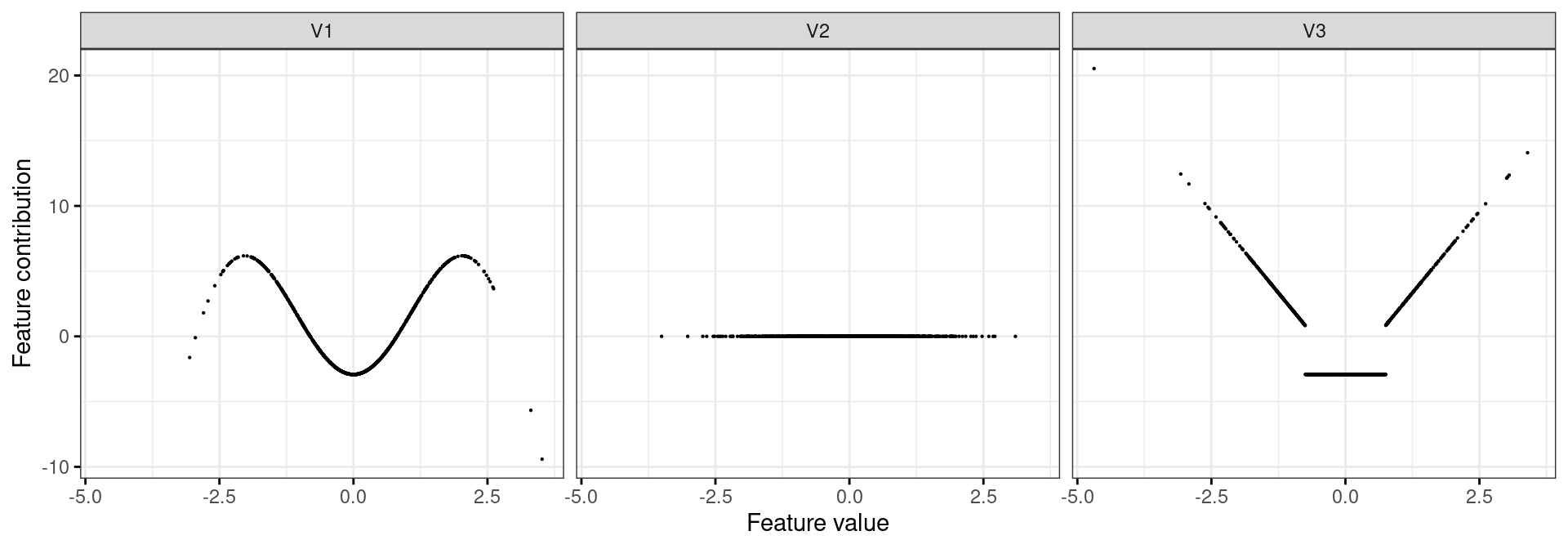## Building a Parameter Encoder NN from scratch

The following code chunks construct a PENN model using keras to estimate beta. The separate functions are combined into a PENN class in PENN.py. I begin by loading the necessary modules below:

# Load backend functions
from keras import backend as b

# Neural net building blocks
from keras.layers import Dense, Input, Lambda, Multiply, Add
from keras.regularizers import l2
from keras import Model

# Necessary for the calculation of the beta-prior
from scipy.spatial import distance_matrix

import tensorflow as tf
# For the loss function to work, we need to switch off eager execution
tf.compat.v1.disable_eager_execution()
# For reproducibility
tf.random.set_seed(42)

Next, I construct the inference network with 2 layers and 10 hidden nodes in each layer. I use a sigmoid activation function. The inference network is completed by the output nodes for the parameter distributions, mu and sigma. The PENN uses variational inference to obtain posteriors of the local parameters. Assuming normally distributed local parameters, mu and sigma parameterize the local posteriors. A prediction is generated by sampling from the posterior:

def build(k, n, mc_draws=100, size=10, l2_penalty=0.001):

# 1. Model inputs

input_inference_nn = Input(k, name='input_inference_nn')
input_model = Input(k, name='input_model')
input_knn_prior = Input(batch_shape=(n, n), name='input_knn_prior')
input_mc = Input(tensor=b.random_normal((n, mc_draws, k)), name='input_mc')
inputs = [input_inference_nn,
input_model,
input_knn_prior,
input_mc]

# 2. Inference Network

encoder_layer_1 = Dense(size,
activation='sigmoid',
kernel_regularizer=l2(l2_penalty))(input_inference_nn)
encoder_layer_2 = Dense(size,
activation='sigmoid',
kernel_regularizer=l2(l2_penalty))(encoder_layer_1)

# ---- Parameter layers
mu = Dense(k, kernel_regularizer=l2(l2_penalty), name='mu')(encoder_layer_2)
sigma_squared = Dense(k, activation='exponential',
kernel_regularizer=l2(l2_penalty))(encoder_layer_2)
sigma = Lambda(lambda i: b.sqrt(i), name='sigma')(sigma_squared)

# 3. Posterior sample

sample = Multiply()([sigma, input_mc])

# 4. Generate predictions

output = Multiply()([sample, input_model])
output = Lambda(lambda i: b.sum(i, axis=2, keepdims=True), output_shape=(n, mc_draws, 1))(output)

# 5. Build model

model = Model(inputs, output)

return model

With the model function defined, I can build the PENN model, as well as supporting models (used only for inference) that extract the parameters of the posterior:

model = build(k, n)

mu_model = Model(model.inputs, model.get_layer('mu').output)
sigma_model = Model(model.inputs, model.get_layer('sigma').output)

The most important component of the PENN is the loss function, which we define next. The loss function consists of two elements: the mean squared error of the local linear model, and a Kullback-Leibler penalty enforcing stability in the parameter distributions:

def loss(y, y_pred):
mse = b.mean(b.square(y_pred - y))
mu_ = model.get_layer('mu').output
sigma_ = model.get_layer('sigma').output
input_knn_prior_ = model.inputs
prior_mu = b.dot(input_knn_prior_, mu_)
prior_sigma = b.dot(input_knn_prior_, sigma_) + b.dot(input_knn_prior_, b.square(mu_ - prior_mu))

kl = b.mean(b.mean((b.log(b.sqrt(sigma_)) -
b.log(b.sqrt(prior_sigma))) -
((sigma_ + b.square(mu_ - prior_mu)) / (2 * prior_sigma)) + 0.5, axis=1))

return mse - kl * lam

Finally, the KNN-prior requires a distance matrix, and we need to set hyperparameters:

lam = 4
gam = 0.04

knn_prior = distance_matrix(x, x)
gam = knn_prior[knn_prior>0.0].min() + gam * (
knn_prior[knn_prior > 0.0].max() - knn_prior[knn_prior>0.0].min()
)
knn_prior /= gam
idx = knn_prior < 1.0
knn_prior[idx] = 1.0
knn_prior[~idx] = 0.0
knn_prior = (knn_prior.T / knn_prior.sum(axis=1)).T

With all the components in place, we can compile and fit the model:

model.compile(loss=loss, optimizer=Adam(learning_rate=0.05, clipnorm=1, clipvalue=0.5))

data = {
'input_inference_nn': x,
'input_model': x,
'input_knn_prior': knn_prior,
'input_mc': np.zeros((n, 100, k))
}
# Note that y needs to be expanded over the sampling dimension (we are sampling 100 draws)
y_expanded = np.repeat(y[:, np.newaxis, np.newaxis], 100, axis=1)

model.fit(data, y_expanded, batch_size=n, epochs=1000, verbose=0)
## <keras.callbacks.History object at 0x7fecdeaeb850>

We can extract the estimated parameters using the inference models:

mu = mu_model.predict(data, batch_size=n)

Let’s plot the posterior means against the values of x: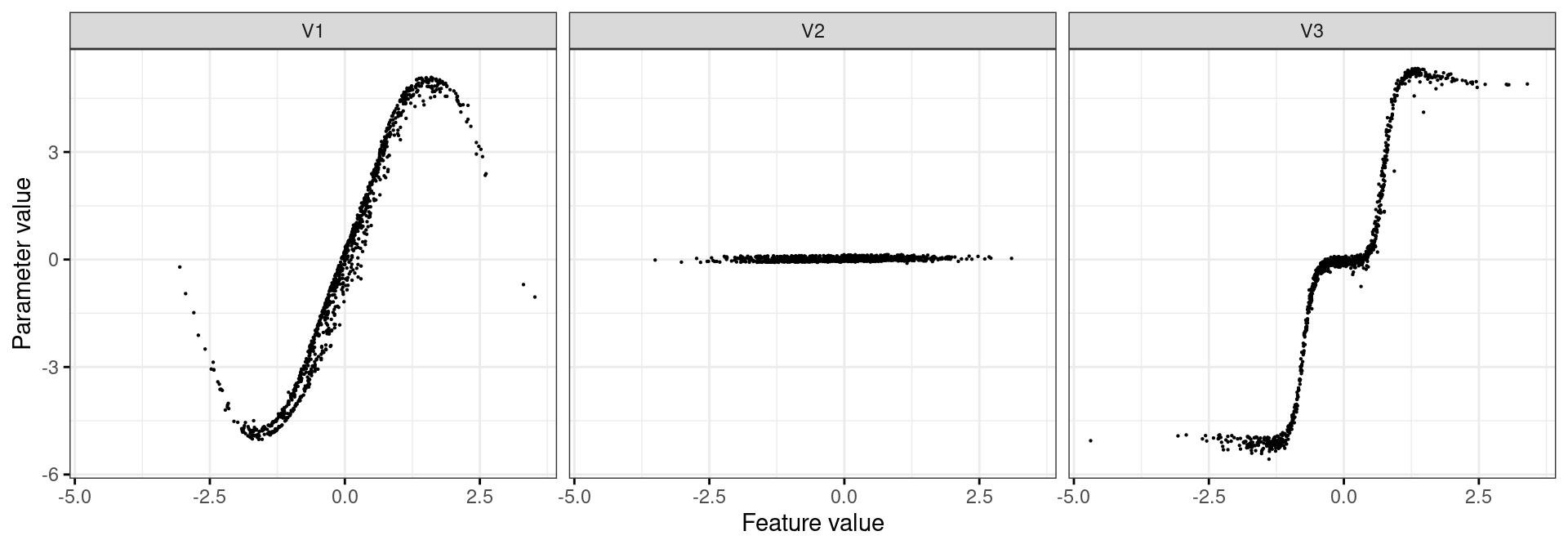…and the true parameters of the simulation against x:That looks pretty good!

## Comparing to shap

An interesting question is how the explanations obtained from the PENN model compare with SHAP values. SHAP values are contributions, which we can obtain from the estimated parameters:

phi = mu * x
phi = phi - phi.mean(axis=0)

Plotting the contributions: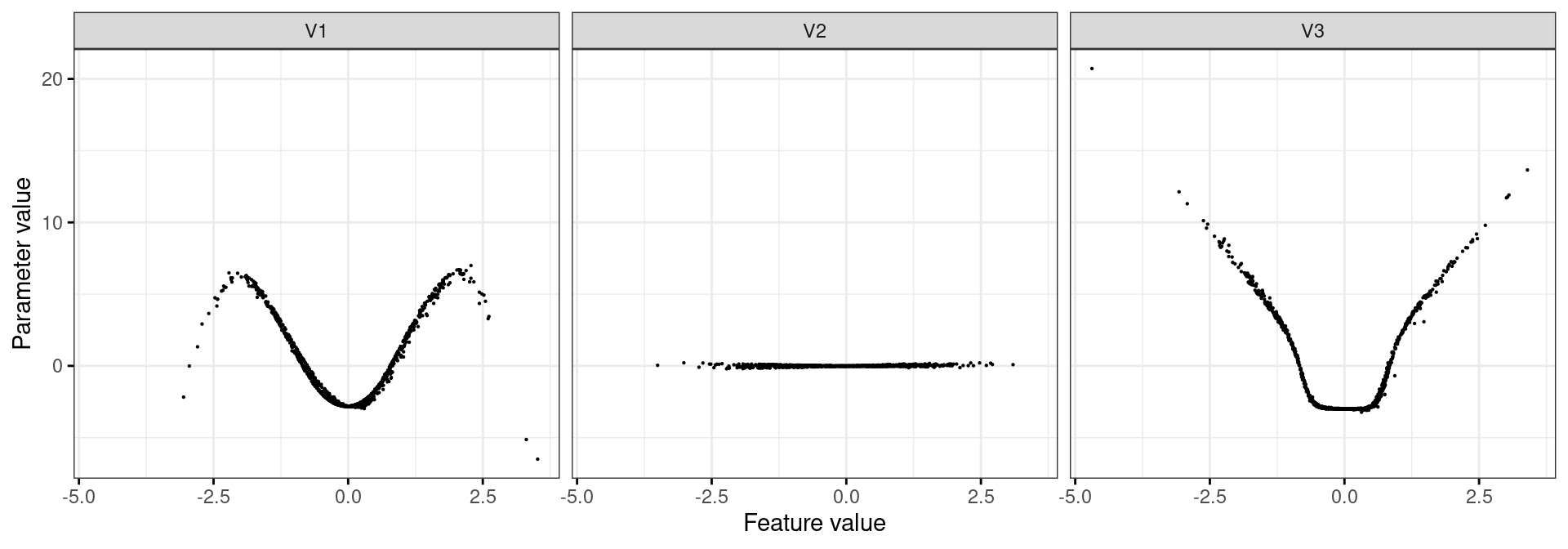Now, I fit a random forest regressor on the data using sklearn:

from sklearn.ensemble import RandomForestRegressor
mod = RandomForestRegressor(n_estimators=100)
mod.fit(x, y)
RandomForestRegressor()
In a Jupyter environment, please rerun this cell to show the HTML representation or trust the notebook.

The feature contributions can be obtained using shap.TreeExplainer:

import shap
expl = shap.TreeExplainer(mod)
phi_shap = expl.shap_values(x, y)

The plot below displays the Random Forest and PENN contributions, which match closely, as is to be expected in the case of independent features: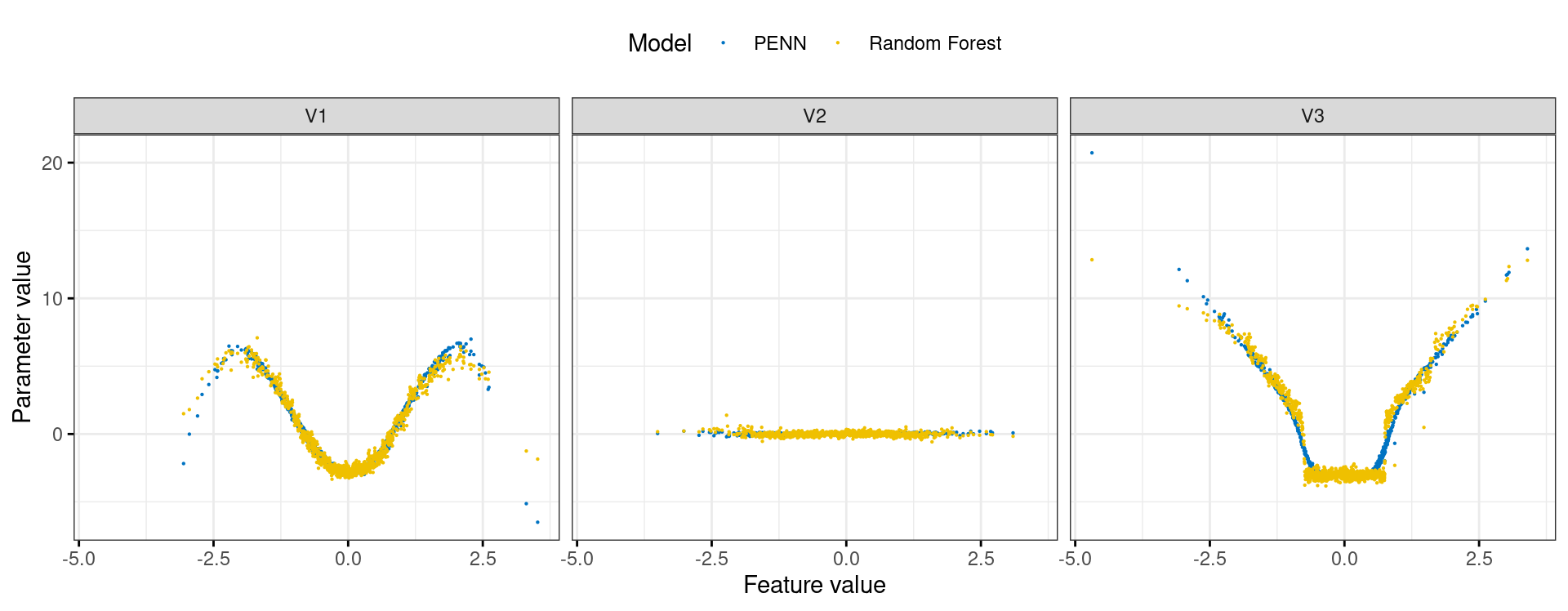The results for the two methods are virtually identical. In a follow-on post, I will show that while this holds for an independent process, in the dependent case, methods such as GAM and SHAP fail.

Pfitzinger, Johann. 2021. “An Interpretable Neural Network for Parameter Inference.” http://arxiv.org/abs/2106.05536.